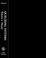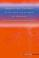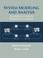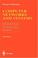### Teletraffic Theory

#### Course Description

Queuing theory - communication network and information traffic applications. Markov processes: basic models of telecommunication traffic. Elementary Markov models. Loss networks, numerical methods for analysis of loss networks. Networks of queues, numerical methods for evaluation of networks of queues. Queuing system analysis. Network traffic modeling. Modeling of voice and video traffic. Tools for traffic analysis of telecommunication networks.

#### General Competencies

Students gain knowledge in the fundamental models of information traffic flows and elementary methods for the traffic analysis, including voice and video traffic. Specifically, students develop a good understanding of the discrete and continuous time Markov chain and its application to different traffic scenarios in packet networks. Students gain a deep understanding of the loss network model applied to the heterogeneous guaranteed services in packet networks and techniques for the capacity sizing.

#### Learning Outcomes

1. identify characteristic of queueing system
2. explain models and methods for analyze queueing systems in communication networks
3. apply simple methods from Traffic theory to communication networks analyzis
4. analyze performance of information and communication systems
5. explain phenomens in different application of queueing theory
6. estimate and evaluate performances of different information and communication systems

#### Forms of Teaching

Lectures

First cycle (seven weeks): lectures then Midterm exam, and Second cycle (six veeks): lectures and Final exam. Lecture duration: 3 hours per week

Exams

Midterm exam: 8th week; Final exam: 15th week.

Consultations

Every week in terms defined by lecturer.

Other Forms of Group and Self Study

Homeworks

By decision of the Faculty Council, in the academic year 2019/2020. the midterm exams are cancelled and the points assigned to that component are transferred to the final exam, unless the teachers have reassigned the points and the grading components differently. See the news for each course for information on knowledge rating.

Continuous Assessment Exam
Mid Term Exam: Written 0 % 50 % 0 %
Final Exam: Written 0 % 40 %
Final Exam: Oral 10 %
Exam: Written 0 % 90 %
Exam: Oral 10 %
##### Comment:

Although homeworks have 0% in a total share in this course, students are obliged to complete them successfully because it is a precondition for passing the exams.

#### Week by Week Schedule

1. Markov processes: elementary models of telecommunication traffic. Discrete-time Markov chains. Continuous-time Markov chains. Birth-death processes. Numerical methods, evaluation of steady-state probabilities, global and local balance equations.
2. Elementary Markov models. Reversibility and Burkes theorem. Evaluation of queuing models M/M/1, M/M/m, M/M/1/k, M/M/m/k, M/M/m/m. Application of Littles theorem.
3. Loss networks. Erlangs model, Engsets model, multiservice loss networks, Kaufman-Roberts recursion.
4. Numerical methods for analysis of loss networks. Jagermans approximation, Labourdette-Hart uniform asymptotic approximation (UAA), reduced load approximation, Kelly and Pascal reduced load approximation.
5. Computer based modeling of telecommunication traffic. The use of Markov chains for modeling traffic, modeling telephone traffic according to Erlang and Engset models, modeling long-range dependent traffic. Tools for traffic analysis of telecommunication networks. NS2 simulator, OPNET simulator and others.
6. Networks of queues. Open and closed queuing networks, product form solution and Jacksons theorem, local and global balance equations, BCMP networks.
7. Numerical methods for evaluation of networks of Markovian queues. Convolution algorithms for closed networks, MVA, approximation and simulation procedures.
8. Midterm examination.
9. Analysis of M/GI/1 and GI/M/1 queuing system. Embedded Markov chain and its application to M/GI/1 and GI/M/1 queuing systems.
10. M/GI/1 queuing systems with priorities. Analysis of the queuing system with the preemptive LCFS queuing discipline, queuing system with HOL service discipline, static priority queuing systems.
11. Network traffic modeling (1). Continuous time and discrete time models: Poisson process, deterministic and Bernoulli process, modulated processes, general interrupted and switched stochastic processes, regressive models, fluid models, ARMA models.
12. Network traffic modeling (2). Self-similarity in real packet networks, self-similar stochastic process, Hurst parameter, physical origins of the self-similarity, sub-exponential distributions, central limit theorem for distributions with infinite variances, a-stable distribution.
13. Network traffic modeling (3). Analysis of real traffic traces and estimation of self-similarity level, Whittle’s estimator, fractal teletraffic models: fractional Brownian noise, fractional ARIMA process.
14. Modeling of voice and video traffic. Voice traffic models based on continuous and discrete time Markov models; autoregressive, Markov, TES (Transform-Expand-Sample) and self-similar models of video traffic.
15. Final examination.

#### Study Programmes

Telecommunication and Informatics (profile)
Theoretical Course (2. semester)

#### LiteratureL. Kleinrock (1975.), Queuing Systems, Volume 1: Theory, Wiley, New York
D. Gross, J.F. Shortle, J.M. Thompson, C.M. Harris (2008.), Fundamentals of Queueing Theory, 4th ed., Wiley, New YorkJ.N. Daigle (2005.), Queuing Theory with Applications to Packet Telecommunication, SpringerH. Kobayashi, B.L. Mark (2009.), System Modeling and Analysis: Foundations of System Performance Evaluation, Pearson-Prentice HallT.G. Robertazzi (2000.), Computer Nertworks and Systems: Queueing Theory and Performance Evaluation?, 3rd ed., Springer
(.), Foundations of Queueig Theory N.U. Prabhu Kluwer 1997,

#### General

ID 34460
Summer semester
5 ECTS
L0 English Level
L1 e-Learning
45 Lectures
0 Exercises
0 Laboratory exercises
0 Project laboratory